# 4.) For the combustion of sucrose: C12H22011+ O2 CO2 H20 There are 10.0 g of sucrose...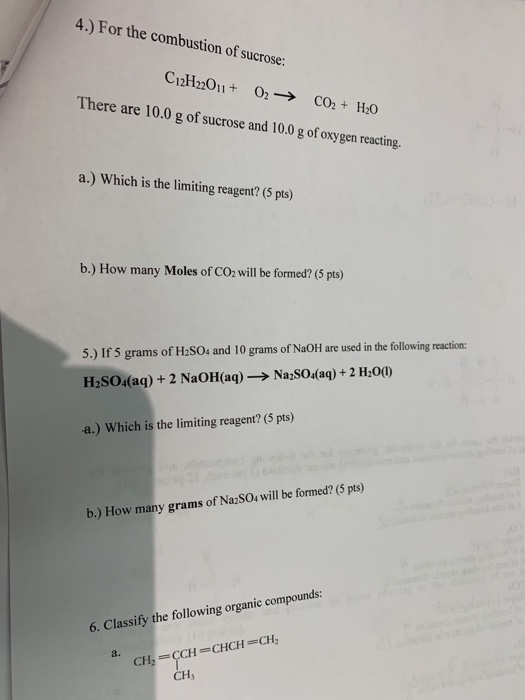4.) For the combustion of sucrose: C12H22011+ O2 CO2 H20 There are 10.0 g of sucrose and 10.0 g of oxygen reacting. a.) Which is the limiting reagent? (5 pts) b.) How many Moles of CO2 will be formed? (5 pts) 5.) If 5 grams of H2SO4 and 10 grams of NaOH are used in the following reaction: Na;SO4(aq) + 2 H2O() H2SO4(aq) + 2 NaOH(aq) a.) Which is the limiting reagent? (5 pts) b.) How many grams of Na2SO4 will be formed? (5 pts) 6. Classify the following organic compounds: a. CH, CCH CHCH CH CH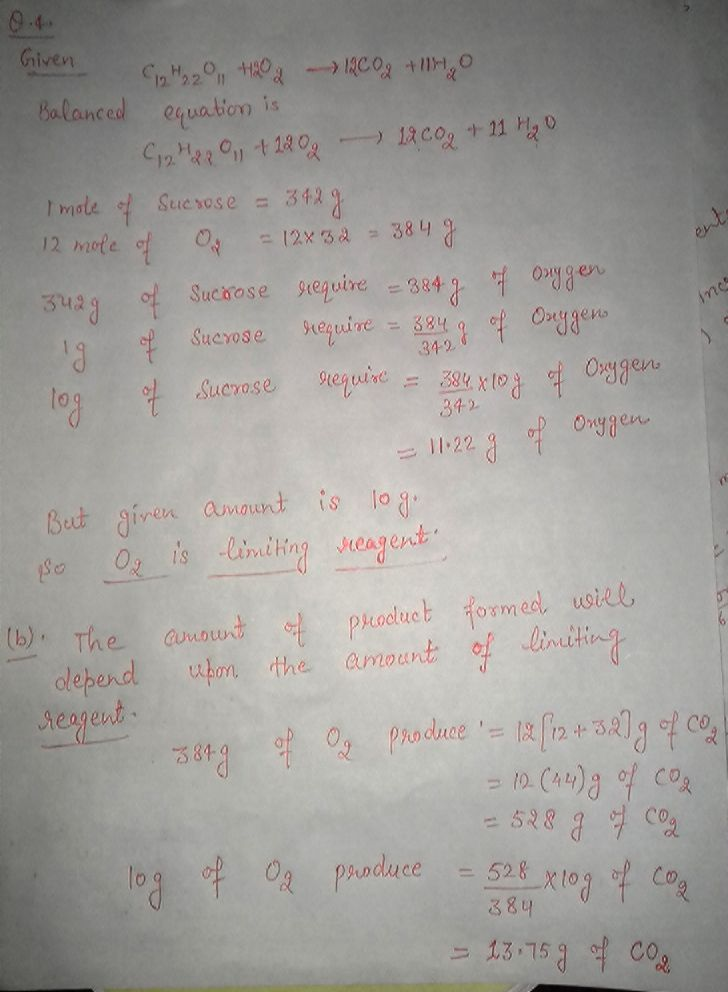5th question solve as 4 th

##### Add Answer of: 4.) For the combustion of sucrose: C12H22011+ O2 CO2 H20 There are 10.0 g of sucrose...
Similar Homework Help Questions
• ### 4. In the reaction below, how many grams of HCl are required to react with 2.000 g of Na,CO,? H20+CO2 () 2 NaCl (a)...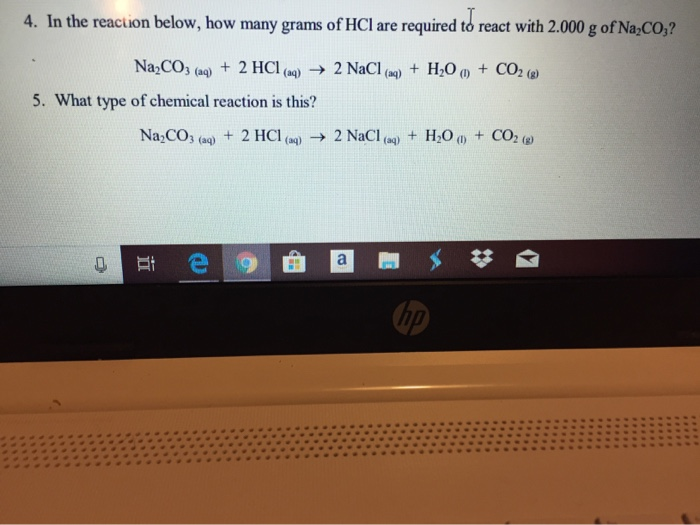4. In the reaction below, how many grams of HCl are required to react with 2.000 g of Na,CO,? H20+CO2 () 2 NaCl (a) Na2CO3 (aq)2 HCl (aq) 5. What type of chemical reaction is this? +CO2 ( Na CO3 (aq) +2 HCl (a) 2 NaCl (a)

• ### Classify each chemical reaction: reaction 4K (s) + O2(g) → 26,0(s) 2CH,CH, (g) + 70, (g) → 400, (g) + 6H120(g) type of...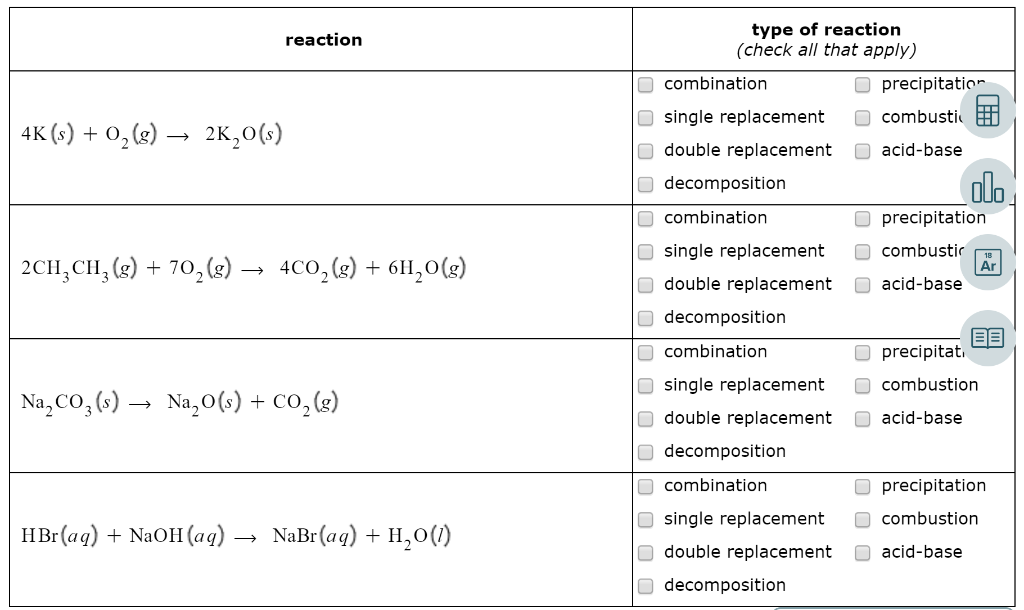Classify each chemical reaction: reaction 4K (s) + O2(g) → 26,0(s) 2CH,CH, (g) + 70, (g) → 400, (g) + 6H120(g) type of reaction (check all that apply) combination precipitation single replacement combusti double replacement acid-base decomposition combination precipitation single replacement combustic double replacement acid-base decomposition combination precipitat single replacement combustion double replacement acid-base decomposition combination precipitation single replacement combustion double replacement acid-base decomposition Na, Coz(s) → Na20(s) + CO2(g) H Br(aq) + NaOH(aq) → NaBr(aq) + H20(1)

• ### Question 37 of 38 > Complete combustion of 5.10 g of a hydrocarbon produced 16.3 g of CO2 and 5.84 g of H20. Wha...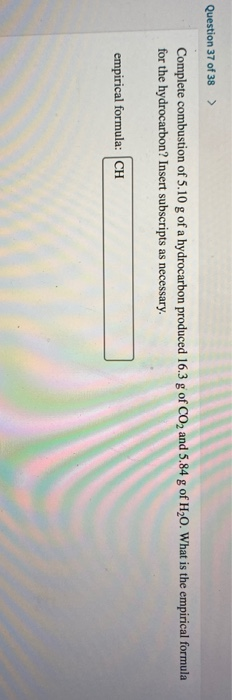Question 37 of 38 > Complete combustion of 5.10 g of a hydrocarbon produced 16.3 g of CO2 and 5.84 g of H20. What is the empirical formula for the hydrocarbon? Insert subscripts as necessary. empirical formula: CH

• ### Question 1 1 pts Calculate the mass of potassium chloride, KCI, (in grams) formed when 7.00 g of chlorine gas is allowe...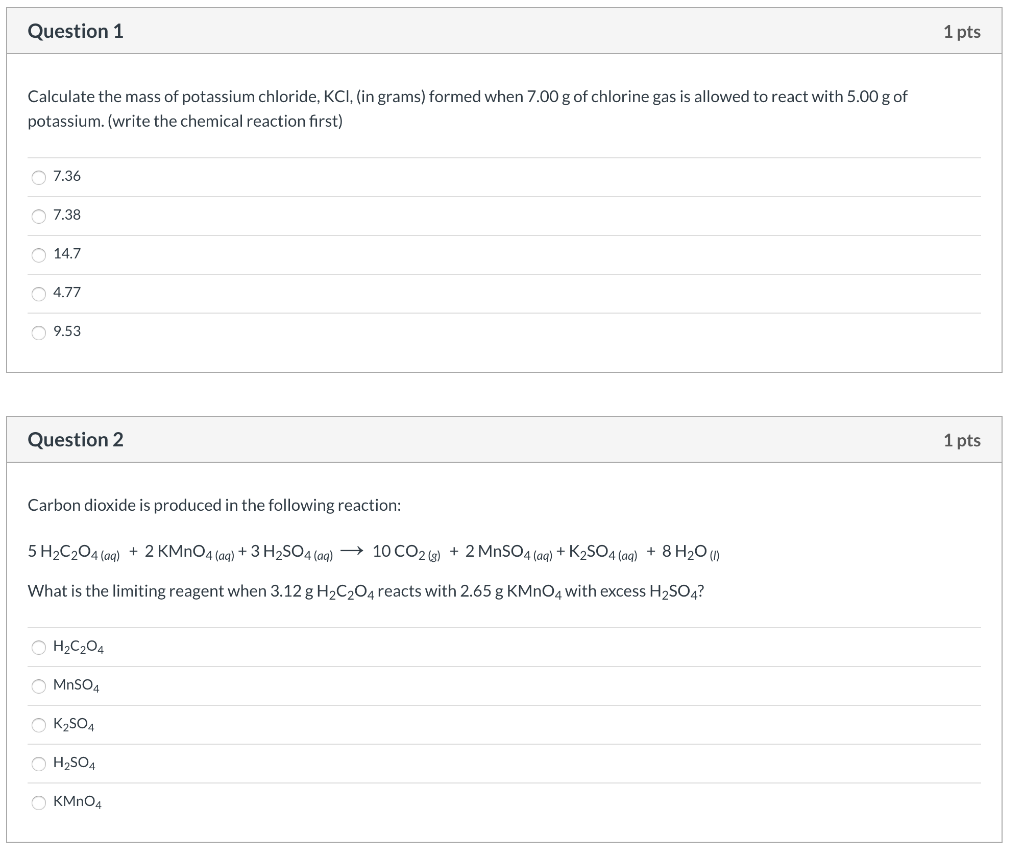Question 1 1 pts Calculate the mass of potassium chloride, KCI, (in grams) formed when 7.00 g of chlorine gas is allowed to react with 5.00 g of potassium. (write the chemical reaction first) 7.36 7.38 14.7 4.77 9.53 Question 2 1 pts Carbon dioxide is produced in the following reaction: 5 H2C204(aq) + 2 KMnO4 (aq) + 3 H2SO4 (aq) → 10 CO2(g) + 2 MnSO4 (aq) + K2SO4 (aq) + 8 H20 (1) What is the limiting reagent...

• ### 1. Consider the reaction: MnO2 (s) + 4 HCl (g)MnCl2 (s) + Cl2 (g) + 2 H20 (g) If 10.00 g of MnO2 ...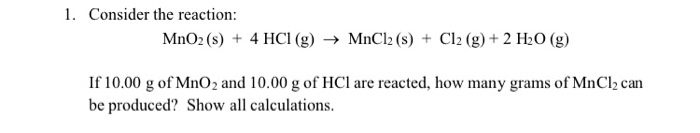1. Consider the reaction: MnO2 (s) + 4 HCl (g)MnCl2 (s) + Cl2 (g) + 2 H20 (g) If 10.00 g of MnO2 and 10.00 g of HCl are reacted, how many grams of MnCl2 can be produced? Show all calculations 2. Consider the following unbalanced equation: NH4NOs(aq) + Na PO4(aq)(NH4)3PO4(aq NaNOs(aq) Assuming you start with 50.0 g of NHNOs and 50.0 g of NasPO4, which is the limiting reagent? What is the theoretical yield (g) of ammonium phosphate? a....

• ### 7. What will the coefficient be for NaOH in the chemical equation listed below when the...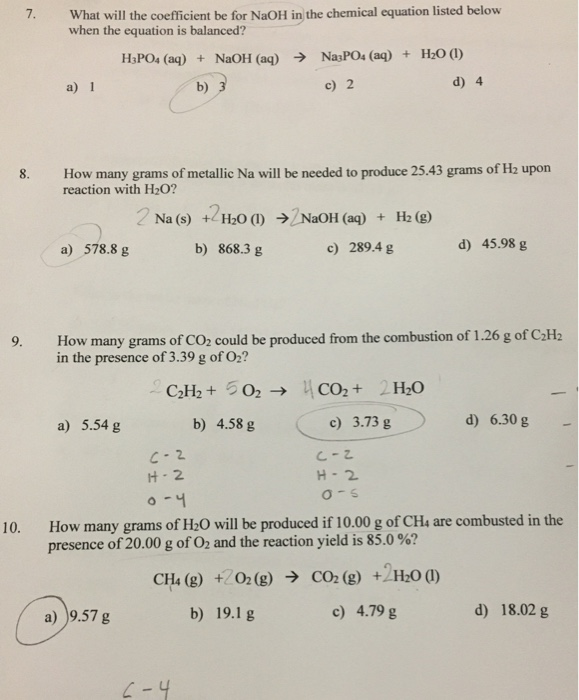7. What will the coefficient be for NaOH in the chemical equation listed below when the equation is balanced? H3PO4 (aq) + NaOH (aq) → NasPO4 (aq) + H20 (1) c) 2 d) 4 How many grams of metallic Na will be needed to produce 25.43 grams of Hz upon reaction with H2O? 2 Na (s) + H20 (1) + NaOH (aq) + H2 (g) a) 578.8 g b) 868.3 g c) 289.4 g d) 45.98 g 9. How many...

• ### Stoichiometry Wor PAGE B) 1Na2SIOs (s)+ 8 HF(aq)H2SiF6 (aq)+ 2 NaF(aq)+3 H20 U) Vh.How many moles...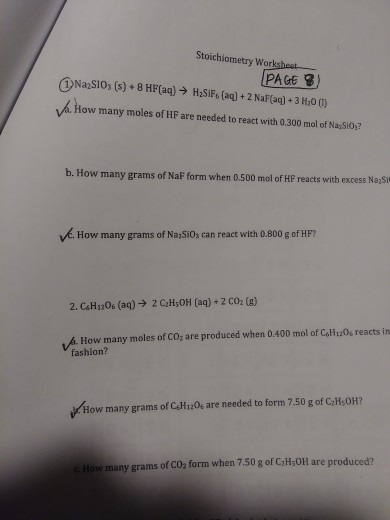Please see attched. Please only do the circle and check questions.1 a, c, 2 a, b Stoichiometry Wor PAGE B) 1Na2SIOs (s)+ 8 HF(aq)H2SiF6 (aq)+ 2 NaF(aq)+3 H20 U) Vh.How many moles of !HIF are needed to react with 0300 mol of NasSi0,? b. How many grams of NaF form when 0.500 mol of HF reacts with excess Na Sit vć. How many grams of Na Si0, can react with 0.800 g of HF7 2. CsH120s (aq) 2 CH,OH [aq)...

• ### 12. Propane (C3H8) is used as a fuel in many gas grills. The balanced equation for the combustion of C3Hg is shown...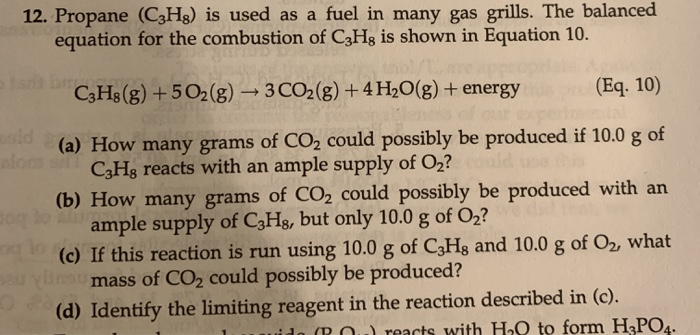12. Propane (C3H8) is used as a fuel in many gas grills. The balanced equation for the combustion of C3Hg is shown in Equation 10. C3H8(g) +502(g) + 3 CO2(g) + 4H2O(g) + energy (Eq. 10) (a) How many grams of CO2 could possibly be produced if 10.0 g of CzHg reacts with an ample supply of Oz? (b) How many grams of CO2 could possibly be produced with an ample supply of C3Hg, but only 10.0 g of O2?...

• ### d. CH 206 to (complete combustion) e. C6H12O6 to — (incomplete combustion) Net Ionic equations can be written...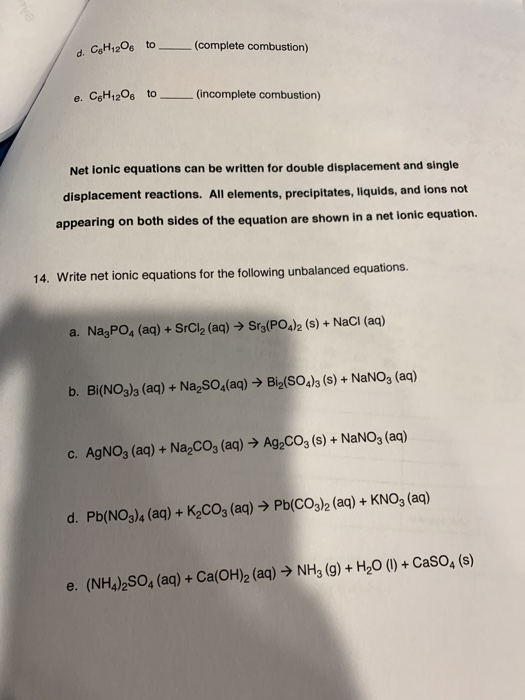d. CH 206 to (complete combustion) e. C6H12O6 to — (incomplete combustion) Net Ionic equations can be written for double displacement and single displacement reactions. All elements, precipitates, liquids, and lons not appearing on both sides of the equation are shown in a net ionic equation. 14. Write net ionic equations for the following unbalanced equations. a. Na3PO4 (aq) + SrCl2 (aq) → Sr3(PO4)2 (s) + NaCl (aq) b. Bi(NO3)2 (aq) + Na, SO4(aq) → Bi2(SO4)3 (s) + NaNO, (aq)...

• ### 1. Classify the following as an endothermic or exothermic reaction: a. Making popcorn in a microwave oven. b. Boili...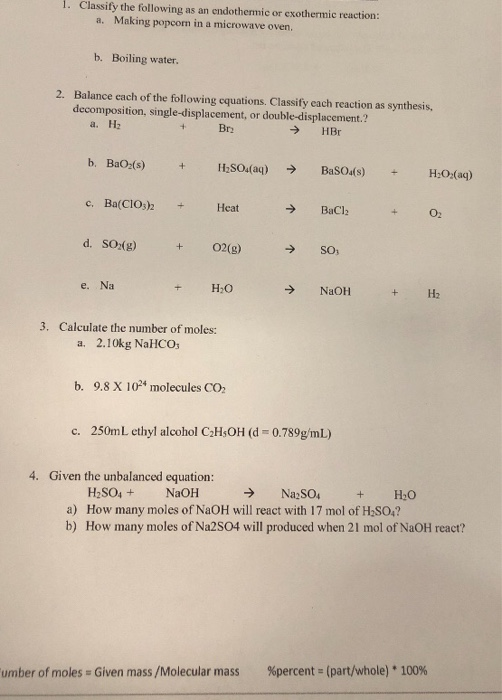1. Classify the following as an endothermic or exothermic reaction: a. Making popcorn in a microwave oven. b. Boiling water. 2. Balance each of the following equations. Classify each reaction as synthesis, decomposition, single-displacement, or double-displacement.? a. H + Bry → HB b. BaOx(s) H2SO4(aq) → BaSO4(s) + H2O2(aq) c, Ba(ClO 2 + Heat → BaCl2 d. Song) + O2(g) → SO, e. Na HO → NaOH + H2 3. Calculate the number of molest a. 2.10kg NaHCO3 b. 9.8...

Need Online Homework Help?